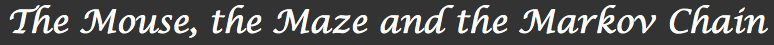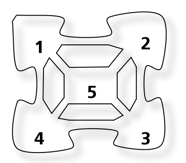1. Introduction2. The mouse and the maze 3. Processes, matrices and Markov chains 4. Will the mouse ever find the exit? How fast? 5. Other examples and applicationsThe mouse and the maze

## 1. Transition ProbabilitiesFigure 1. The maze
Little Ricky is a mouse and she lives in a small maze where she likes to wander from room to room. The layout of her living space is depicted in figure 1.

Every thirty minutes or so she likes to change rooms and just moves to one of the rooms adjacent to the one she is in at that moment. For example, from room number 1, she might decide to move on to rooms number 2, 4 or 5, but she wouldn’t go all the way to room 3. She is a lazy rodent after all.

A careful study of her habits shows that the room she decides to go to next does not depend on any kind of history. She just seems to pick one the rooms she has access to (excluding her current location) randomly, where each accessible room is equally likely to be chosen.

In this setting, given an initial room i for the mouse to be in – i can be equal to 1, 2, 3, 4 or 5 – and for each other room j , there is a well defined number that represents the probability for the mouse to move from room i to room j.

Notice that this number does not depend on the time at which we evaluate that probability nor does it depend on what other rooms the mouse visited before that.

Definition
If the mouse is inside room i, we will say it is in the state i. We then define the transition probability pji to be the probability for the mouse to move to room j if she starts in room i.

For example, here p21 = 1/3 being the probability for the mouse to go from room 1 to room 2: she has access to 3 rooms (2, 4 and 5) all of which are equally likely, hence the value 1/3. In our example, she can’t stay in the same room more than 30 minutes, so p11 = 0 for example, meaning that the probability that she stays in room 1 starting in room 1 is 0.

Question
Find all the transition probabilities pji for and i and j ranging from 1 to 5.
Question
What can you say about the sum p21 + p41 + p51 ? Is this a surprise? How about p12+ p15+ p14? Can you explain these results?

Remember that if Ω = {ω1, ..., ωn } is probability model, where the ωi ’s are the possible future events or outcomes, and if pi is the probability of the event ωi occurring, then p1 + p2 + ... + pn =. This just says that the sum of all probabilities is equal to on, as something has to happen.

For example the mouse deciding to move from room 1 to room 2 is one of the outcomes in the probability model describing the mouse in room 1, and its probability is 1/3 as calculated before.

## 2. Steps and Probabilities

Let us put Little Ricky back into room number 1 and watch what happens. After about 30 minutes, she moves to another room. This could be room 2, room 4 or room 5.

After yet another 30 minutes, she moves again to one of the adjacent rooms, and so on. For example, she might decide to move on to room 2 and then to room 3. But she might also want to come back to room 1 after she visits room 2.

Question
Suppose Little Ricky starts in room 1 and then moves to room 2. What is the probability of her returning to room 1 next time she moves? Remember that we assumed that she moved from to room independently of any kind of history and always following the transition probabilities that we defined earlier.
Question
Suppose she first moves to room 5, instead of 2. What is now the probability of her returning to room 1 at the next step?
Question
Starting from room number 1 now. Can you calculate the probability of her returning to that room after 2 steps? How about after 3?

Let us try and solve this problem for 2 steps. To simplify the notation, we will write R0 to denote the room she is in at the beginning of the experiment, R1 to denote the room she first moves to, an so on. In this language we are trying to calculate the probability of {R0 = 1 and R2 = 1}. Call this event X

Now this event can be broken apart into 3 events depending on where she first moves to: A = {R0 = 1 and R1 = 2 and R2 = 1}, B = {R0 = 1 and R1 = 4 and R2 = 1 } and C = {R0 = 1 and R1 = 5 and R2 = 1}. By broken apart we mean that in our probability model the event X is equivalent to A or B or C . Mathematically, this means:

X = ABC

In general, calculating the probability P(AB) of A or B happening is given by P(AB) = P(A) + P(B) − P(AB), where the last term is the probability of both A and B happening. But when A and B are disjoint, meaning they can not happen at the same time, this probability simply becomes P(A) + P(B). In our case, the mouse can’t be in both rooms 2 and 4 after the first step, for example. The events A, B and C and are all mutually disjoint, and thus we get:

P(X ) = P(A) + P(B) + P(C )

While moving from one value to calculate to three may seem like making the problem only harder, it is the very opposite in reality. Let us look at P(A), for example.

The event A occurs when Little Ricky first moves to room number 2, and then to room number 1 again. The probability of A occurring is thus the probability of her moving from room 1 to room 2 at the first move, and from room 2 to room 1 at the second one.

Now remember that we assumed that she moved from room to room independently of any kind of history. This means that her moving from room 1 to room 2 at first, and her moving from room 2 to room 1 at the second step are two independent events. Probability theory tells us that in a case like this, where Y and Z are two independent events, the probability of both Y and Z happening is simply the product of their probabilities, that is to say P(Y ∩ Z) = P(Y)P(Z).

Putting everything together, we get P(A) = P(moving from room 1 to room 2 at step 1) ∗ P(moving from room 2 to room 1 at step 2). Remembering that those probabilities do not depend on the time and are the transition probabilities pij this simplifies to:

P(A) = p12 ∗ p21

Question
Carry the same process and show that P(B) = p14 p41 and that a similar expression holds for P(C ).

We have now shown that:You might now be able to guess the probability of Little Ricky ending up in room 2 after 2 steps, say. Indeed, just break that even apart depending on what room she first moves 2, then calculate the 3 probabilities using the same principles we used in the previous example.

Question
Calculate the probability of Little Ricky ending up in room 2 after 2 steps starting from room 1.
Question
How about the probability of ending up in room 2 starting from 1 after 3 steps? 4 steps? n steps?

While you should be able to carry on this analysis in theory, the calculations might quickly get out of control in practice. The framework we will introduce in the next section will help solve that problem and make a lot of these calculations much simpler, so that they can even ben carried on by a computer or graphing calculator. This is only a hint of why the Markov chain framework is so popular. Before we do that, we dedicate the next subsection to introducing another important concept.

## 3. States of Mice

Suppose we first flip a coin then put Little Ricky in room 1 or 2 depending on what the outcome is. For example, we put Little Ricky in room 1 if the coin lands on tails and in room 2 otherwise. One might wonder what the probability of Little Ricky ending up in room 5 after 1 step would be, and we will show to find that out. We assume that the coin is fair so that both side are equally likely to come out, with a probability of 1/2 , and that the coin and the mouse are ”independent” of one another.

Let then X be the event ”she ends up in 5 after 1 step”, then following the same kind of analysis we did earlier, we can break this event apart into two events: A = {she ends up in 5 starting from 1} and B = {she ends up in 5 starting in 2}.

Question
Explain why P(X) = P(A) + P(B). Now the event A is equivalent to {She moves from 1 to 5} and {The coin landed on tail}.
Question
Show that P(A) = (1/2) p15 . Remember that the mouse and the coin are independent.
Question
Conclude that P(X) = (1/2) p51 + (1/2) p52

Let us now assume that we roll an unfair 5-sided die such that the side i comes out the probability qi . We do not assume the die to be fair, so we do not require q1 = q2 = ... = q5 . On the other hand, the qi are probability values so they are all non-negative and satisfy q1 + q2 + ... + q5 = 1.

Suppose we roll the die and drop Little Ricky in the room whose number comes out. In other words, she might be dropped in room 1 with probability q1, or in room 2 with probability q2, and so on.

Question
What is the probability of her ending up in room 5 after 0 steps?
Question
What is the probability of her ending up in room 5 after 1 step? To answer this question, break this event apart again depending on where she is dropped first. You should get
p51 q1 + p52 q2 + p53 q3 + p54 q4 + p55 q5                 (3)
Question
Can you do the same calculation for 2 steps? Use what we’ve calculated earlier.